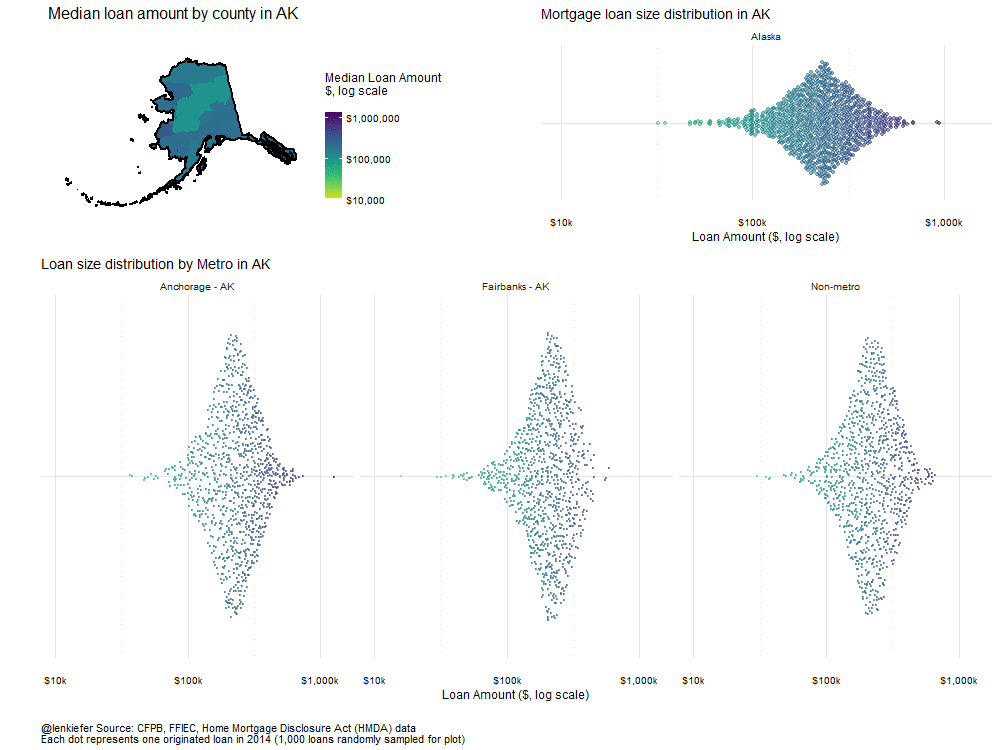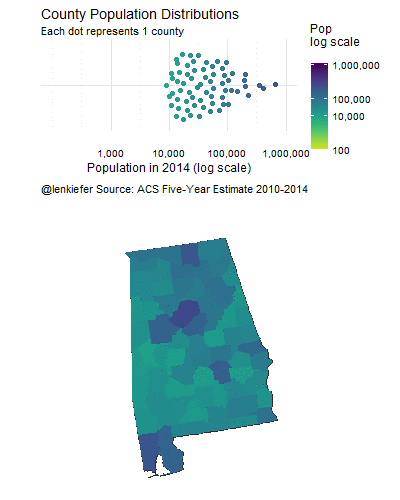Maps, mortgages and me

IN THIS POST I WANT TO DOCUMENT some R code I’ve recently been working on combining maps and distribution plots. As I discussed earlier lots of interesting data will be released in the fall and I want to be ready for it.

Some of these snippets can be recycled when the new data is available.

Maps

One area of data visualization with R I haven’t explored much is mapping. Part of this reason is because I’ve had other tools to use, but usually it’s because I’m in a hurry. Maybe because of prior experience with SPlus and the way ggplot is structured I’ve found making other statistical graphs (line charts, scatters, etc.) relatively easy in R. But maps have been a different story.

Because my mapping interests are relatively simple (I’m usually just looking for a choropleth), I’ve been able to get by with other tools like Tableau or even SAS. For examples, see this post, this post, this post or my Tableau profile.

But there is a lot of cool stuff being done in R with maps. I have found recent posts by Kyle Walker and Julia Silge to be very helpful to get me started. For example this post by Kyle and this one by Julia have been my launching points for this analysis. I’m sure there are lots of other people doing interesting stuff–please tell me about it–but those two articles were useful for relative beginners such as myself.

For this post I’m going to return to the 2014 Home Mortgage Disclosure Act Data (HMDA) that you can get from the Consumer Financial Protection Bureau (CFPB) webpage and I discussed earlier.

The goal will be to create a choropleth map showing some summary statistics of the HMDA data and to integrate it with some other statistical analysis. One example we’ll try to build is this graph I posted on Twitter last night:

If you haven’t already go ahead and click on the follow button.

The map in the upper left corner is a choropleth map showing the median loan size by county for mortgage loans originated in California in 2014. The other charts are beeswarm plots showing the distribution of loan size for California as a whole (upper right corner) and for each of the metro areas (and a non-metro residual) located in California.

Building the maps

I’m going to be adapting the code from Julia’s post to make this map.

First let’s get the data we need. I’m going to be using the same HMDA data from before that you can download from the CFPB via this link.

The HMDA data in the .csv file includes state and county names, but not Federal Information Processing Series (FIPS) codes. FIPS codes are convenient for working with geographic data. Names are sometimes formatted differently (do they include the word “county” for example) so having FIPS codes would make our life easier. Fortunately the Census has a convenient lookup file we can use. This or a related file might well be included somewhere in some R package, even one I use, but didn’t see it.

{% highlight r #load libraries library(‘ggbeeswarm’) library(data.table) library(ggplot2) library(scales) library(ggthemes) library(acs) library(reshape2) library(stringr) library(ggthemes) library(ggalt) library(rgeos) library(maptools) library(albersusa) library(broom) library(dplyr) library(tweenr) library(purrr) library(animation) library(viridis)

#load data and scripts mydata <- fread(”~/data/hmda_lar.csv”) source(’~/code/multiplot.R’)

function for combining graphs see: http://www.cookbook-r.com/Graphs/Multiple_graphs_on_one_page_(ggplot2)/

#data stuff

#trim columns off data mydata<-mydata[,list(state_name,state_abbr,county_name,loan_amount_000s,loan_purpose_name,loan_type_name, applicant_income_000s,lien_status_name,msamd_name)]

#create merged state + county variable mydata<-mydata[, c.name:=str_c(state_abbr,”:“,county_name)]

#get fips lookup: from census

fips.look<-fips.look[,fips := str_c(str_pad(st.fips, 2, “left”, “0”),str_pad(county.fips, 3, “left”, “0”))] #create merged state + county variable fips.look<-fips.look[,c.name:=str_c(state_abbr,”:“,county_name)] #get rid of extra columns for merge fips.look2<-fips.look[,list(fips,c.name)]

#merge fips numbers back onto data mydata<-merge(mydata,fips.look2,by=“c.name”)

#add state code, will be useful for labeling mydata<-mydata[,st.fips:=substr(fips,1,2)] mydata<-mydata[,county.fips:=substr(fips,3,5)]

loan amounts read as character variable, scaled in $1000s, create upb variable in$s and numeric

mydata$upb<-as.numeric(mydata$loan_amount_000s)*1000

Create a summary file that has total UPB (upb), median loan amount (upb.med), and count of loans (count)

county.sum<-mydata[,list(upb=sum(upb),upb.med=median(upb),count=.N), by=list(fips,state_abbr,state_name,county_name,msamd_name)] {% endhighlight Now that we’ve loaded our data and summarized it, let’s load some maps.

{% highlight r

Let’s load some maps:

states<-usa_composite() #create a state map thing smap<-fortify(states,region=“fips_state”) smap.all<-smap #we’re going to subset smap later, so copy full map

counties <- counties_composite() #create a county map thing

#add on summary stats by county using FIPS code counties@data <- left_join(counties@data, county.sum, by = “fips”)
cmap <- fortify(counties_composite(), region=“fips”) #create state and county FIPS codes cmap$state<-substr(cmap$id,1,2)

The next step is to make a function that generates the composite plot based on a state FIPS number:

myplot<-function(i){

c.list<-unique(mydata[st.fips ==st.list[i]]$fips) # all counties within selected state [i] smap<-subset(smap.all, id %in% st.list[i]) # subset state map cmap<-subset(cmap.all, fips %in% c.list) # subset county map #state label st.label<-unique(fips.look[st.fips==as.numeric(st.list[i])]$state_abbr)

graph 1: map (as above, but only including subset)

g1<- ggplot() + geom_map(data = cmap, map = cmap, aes(x = long, y = lat, map_id = id), color = “#2b2b2b”, size = 0.05, fill = NA) + geom_map(data = counties@data, map = cmap, aes(fill =log(upb.med), map_id = fips), color = NA) + geom_map(data = smap, map = smap, aes(x = long, y = lat, map_id = id), color = “black”, size = 1.05, fill = NA) + theme_map( base_size = 12) + theme(plot.title=element_text( size = 16, margin=margin(b=10))) + theme(plot.subtitle=element_text(size = 14, margin=margin(b=-20))) + theme(plot.caption=element_text(size = 9, margin=margin(t=-15),hjust=0)) + coord_proj(us_laea_proj) + labs(y=“Loan Amount, $”,x=“Loan Purpose”, title=paste(“Median loan amount by county in”, unique(fips.look[st.fips==as.numeric(st.list[i])]$state_abbr)))+ scale_fill_viridis(name=“Median Loan Amount\n$, log scale\n”, discrete=F,option=“D”,end=0.95,direction=-1,limits=c(log(10000),log(1.4e6)), breaks=c(log(10000),log(100000),log(1e6)), labels=c(“$10,000”,“$100,000”,“$1,000,000”) )+ theme(legend.position = “right”)

#plot data:

Prepare data: select only data in the state (derived from c.list)

pdata<-county.sum[fips %in% c.list]

pdata2<-mydata[fips %in% c.list,.SD[sample(.N,min(.N,1000))],by = msamd_name ] #subsample metro data

http://stackoverflow.com/questions/27325656/how-do-you-sample-groups-in-a-data-table-with-a-caveat

pdata2[msamd_name==“”,msamd_name:=“Non-metro”] #rename missing metros to “Non-Metro”

pdata2[,.N,by=msamd_name] # Can run this to check how many obs we have per metro

pdata3<-mydata[fips %in% c.list][sample(.N,1000)] #subsample state data

g2<- ggplot(data=pdata2,aes(y=“”,x=upb,color=log(upb)))+ geom_quasirandom(alpha=0.5,size=0.35)+ theme_minimal()+ scale_color_viridis(name=“Loan Amount\n$,log scale\n”,discrete=F,option=“D”,end=0.95,direction=-1, limits=c(log(10000),log(1.4e6)), breaks=c(log(10000),log(100000),log(1e6)), labels=c(“$10k”,“$100k”,“$1,000k”) ) + scale_x_log10(limits=c(10000,1.4e6),breaks=c(10000,100000,1000000), labels=c(“$10k”,“$100k”,“$1,000k”) )+ theme(plot.title=element_text(size=14))+theme(plot.caption=element_text(hjust=0,vjust=1,margin=margin(t=10)))+ theme(plot.margin=unit(c(0.25,0.25,0.25,0.25),“cm”))+ theme(legend.position = “none”)+ labs(y=“”,x=“Loan Amount ($, log scale)”, caption=”\n@lenkiefer Source: CFPB, FFIEC, Home Mortgage Disclosure Act (HMDA) data\nEach dot represents one originated loan in 2014 (1,000 loans randomly sampled for plot)“, title=paste(“Loan size distribution by Metro in”, unique(fips.look[st.fips==as.numeric(st.list[i])]$state_abbr)))+ theme(axis.text.x = element_text(size=4))+ facet_wrap(~msamd_name)+theme(strip.text.x = element_text(size = 4)) graph 3: upb distribution by county (using a subsample of 1000 obs) g3<- ggplot(data=pdata3,aes(y=“”,x=upb,color=log(upb)))+geom_quasirandom(alpha=0.5,size=0.75)+ theme_minimal()+ scale_color_viridis(name=“Loan Amount\n$,log scale\n”,discrete=F,option=“D”,end=0.95,direction=-1, limits=c(log(10000),log(1.4e6)), breaks=c(log(10000),log(100000),log(1e6)), labels=c(“$10k”,“$100k”,“$1,000k”) ) + scale_x_log10(limits=c(10000,1.4e6),breaks=c(10000,100000,1000000), labels=c(“$10k”,“$100k”,“$1,000k”) )+ theme(plot.title=element_text(size=14))+theme(plot.caption=element_text(hjust=0,vjust=1,margin=margin(t=10)))+ theme(plot.margin=unit(c(0.25,0.25,0.25,0.25),“cm”))+ theme(legend.position = “none”)+ labs(y=“”,x=“Loan Amount ($, log scale)”, title=paste(“Mortgage loan size distribution in”, unique(fips.look[st.fips==as.numeric(st.list[i])]$state_abbr)))+ facet_wrap(~state_name)

m<-multiplot(g1,g2,g3,layout=matrix(c(1,3,2,2,2,2), nrow=3, byrow=TRUE))

}

Try it out for California:

myplot(5) {% endhighlightAnimated gif

We can loop through all states and make a gif out of it:

{% highlight r oopt = ani.options(interval = 1) saveGIF({for (i in 1:51) { g<-myplot(i) print(g) ani.pause() print(i) } },movie.name=“HMDA loan amounts v4.gif”,ani.width = 1000, ani.height = 750) {% endhighlightCounty Population Example

I began figuring out make maps in R by replicating Julia Silge’s excellent example. Then I combined tweenr and beeswarm plots to show how county population is distributed across states:Code for county population example

Once again we’ll use the R package tweenr and animate to make an animated gif. See my earlier post about tweenr for an introduction to tweenr, and more examples here and here.

{% highlight r

You’ll need api key & acs package (see julia’s post)

countygeo <- geo.make(state = “”, county = “”) popfetch <- acs.fetch(geography = countygeo, endyear = 2014, span = 5, table.number = “B01003”, col.names = “pretty”) myfips <- geography(popfetch) %>%
mutate(fips = str_c(str_pad(state, 2, “left”, “0”), str_pad(county, 3, “left”, “0”))) %>% select(fips) geography(popfetch)=cbind(myfips, geography(popfetch)) popDF <- melt(estimate(popfetch)) %>% mutate(fips = str_sub(str_c(“00”, Var1), -5), pop2014 = value) %>% select(fips, pop2014) head(popDF)

We use the sampe cmap, though we merge population data instead of mortgage data

counties <- counties_composite() counties@data <- left_join(counties@data, popDF, by = “fips”) cmap <- fortify(counties_composite(), region=“fips”) cmap$state<-substr(cmap$id,1,2) cmap.all<-cmap cmap<-subset(cmap.all, state==st.list)

popDF$state<-substr(popDF$fips,1,2) st.list<-unique(popDF$state) popDF<-data.table(popDF) all_states <- map_data(“state”) st.name<-unique(all_states$region) #get a list of states

popDF$fips<-factor(popDF$fips) popDF$state<-factor(popDF$state)

pause for discussion

{% endhighlight

In order to get the tweenr animation to work, I need to ensure that each data frame I feed to the tween_states() function has the same number of observations. But states have different numbers of counties. Texas has the most with 254 counties.

We’ll set up a blank data frame based on Texas and index the county number. We’ll merge each state to this data frame, which will give us missing values for county numbers less than 254.

{% highlight r popDF<-data.table(popDF) blank.df<-popDF[state==“48”,] #max number of counties is Texas at 254
blank.df[,idn:=.I] #create index

popDF<-popDF[ , idn := 1:.N , by = state ] #now create index for each county by state

function to create data set for animated swarm plot

myf<-function(mystate){ temp<-merge(blank.df[,list(idn)], popDF[state==mystate,], by=“idn”,all.x=T) temp$fips<-factor(temp$fips) temp$state<-factor(temp$state) temp$idn<-factor(temp$idn) temp<-temp[order(idn)] return(data.frame(temp)) }

graph 1: set up animated beeswarm

g1<- ggplot(dtf[.frame==i], aes(y=“”,x=pop2014,color=log(pop2014)))+ theme_minimal()+
theme(plot.title=element_text(size=14))+theme(plot.caption=element_text(hjust=0,vjust=1,margin=margin(t=10)))+ theme(plot.margin=unit(c(0.25,0.25,0.25,0.25),“cm”))+ theme(legend.position = “right”)+ geom_quasirandom(alpha=0.95,size=2) + #I like the quasirandom beeswarm option scale_color_viridis(name=“Pop\nlog scale\n”, discrete=F,option=“D”,end=0.95,direction=-1,limits=c(log(100),log(1e7)), breaks=c(log(100),log(10000),log(100000),log(1e7)), labels=c(“100”,“10,000”,“100,000”,“1,000,000”)) + scale_x_log10(label=comma,limits=c(100,1e6),breaks=c(1000,10000,100000,1000000))+ labs(x=“Population in 2014 (log scale)”,y=“”, subtitle=“Each dot represents 1 county”,title=paste(st.label,“County Population Distributions”), caption=“@lenkiefer Source: ACS Five-Year Estimate 2010-2014”)

graph 2: set up map

fips.c<-as.character(head(dtf[.frame==i]\$state,1)) #get fips code for iteration i cmap<-subset(cmap.all, state==fips.c)

g2<- ggplot() + geom_map(data = cmap, map = cmap, aes(x = long, y = lat, map_id = id), color = “#2b2b2b”, size = 0.05, fill = NA) + geom_map(data = counties@data, map = cmap, aes(fill = log(pop2014), map_id = fips), color = NA) + theme_map( base_size = 12) + theme(plot.title=element_text( size = 16, margin=margin(b=10))) + theme(plot.subtitle=element_text(size = 14, margin=margin(b=-20))) + theme(plot.caption=element_text(size = 9, margin=margin(t=-15))) + coord_proj(us_laea_proj) + labs(title=“”,subtitle=“” ) + scale_fill_viridis(name = “Population”, discrete=F,option=“D”,end=0.95,direction=-1)+ theme(legend.position = “none”)

m<-multiplot(g1,g2,layout=matrix(c(1,1,2,2,2), nrow=5, byrow=TRUE)) print(m) print(i) ani.pause() } for (i2 in 1:2) {print(m) ani.pause() } },movie.name=“map and dot v2.gif”,ani.width = 400, ani.height = 500) {% endhighlight

What hasn’t worked out so well (yet)

I’ve got a few other ideas that haven’t quite worked out. You can learn a lot from failures (especially your own), so I’ll include an example.

I wanted to compare the distribution of county population across states to the U.S. as a whole. So I thought I’d use a beeswarm plot (with geom_quasirandom). I wanted the state distribution to literally fall out of the national distribution using an animation. I got this far:Technically I had some trouble getting the labels on the plot (which state is dropping), but I think there’s more wrong with it than that. Maybe we can fix it up for a later post.